Скачать презентацию Announcements 10 11 10 Prayer n Exams not graded all

• Количество слайдов: 18Announcements 10/11/10 Prayer n Exams… not graded all the way yet, hopefully by tomorrow. n Matt Allen, Jake Peery: Are you present? See me ASAP. n HW notes: – As said in email: must turn in actual printouts when problem says, “Use a computer program such as Mathematica…” – As said in later email: if can’t get printer to work, can send plots to grader via email (Chris’s email address is on class website) – As said in still later email: “how to print” instructions given, so try those before sending plots to Chris by email. n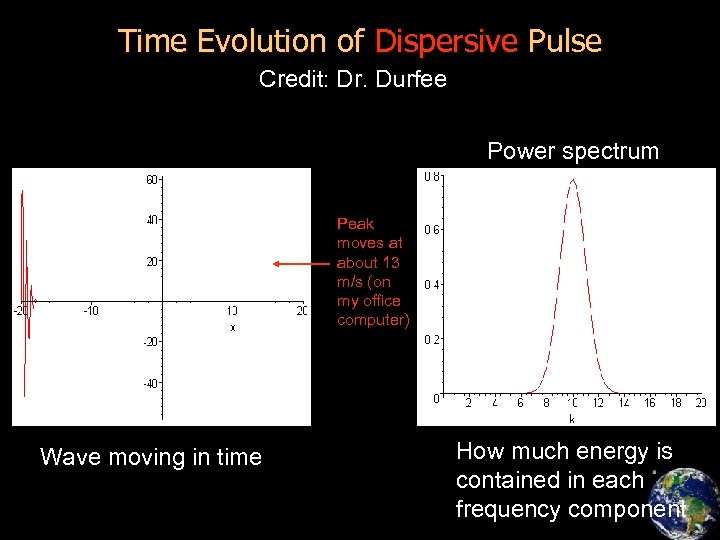Time Evolution of Dispersive Pulse Credit: Dr. Durfee Power spectrum Peak moves at about 13 m/s (on my office computer) Wave moving in time How much energy is contained in each frequency component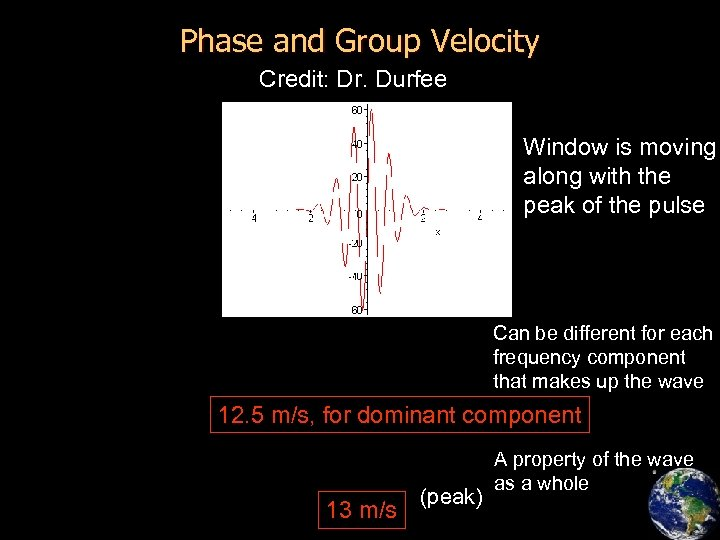Phase and Group Velocity Credit: Dr. Durfee Window is moving along with the peak of the pulse Can be different for each frequency component that makes up the wave 12. 5 m/s, for dominant component 13 m/s (peak) A property of the wave as a whole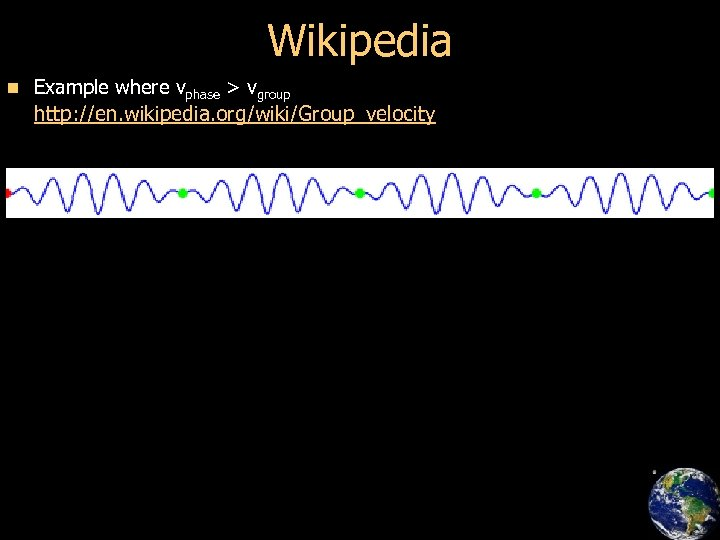Wikipedia n Example where vphase > vgroup http: //en. wikipedia. org/wiki/Group_velocity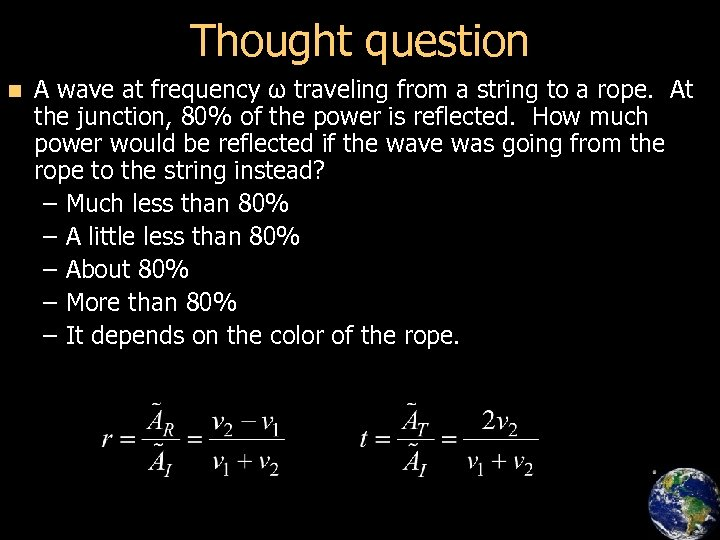Thought question n A wave at frequency ω traveling from a string to a rope. At the junction, 80% of the power is reflected. How much power would be reflected if the wave was going from the rope to the string instead? – Much less than 80% – A little less than 80% – About 80% – More than 80% – It depends on the color of the rope.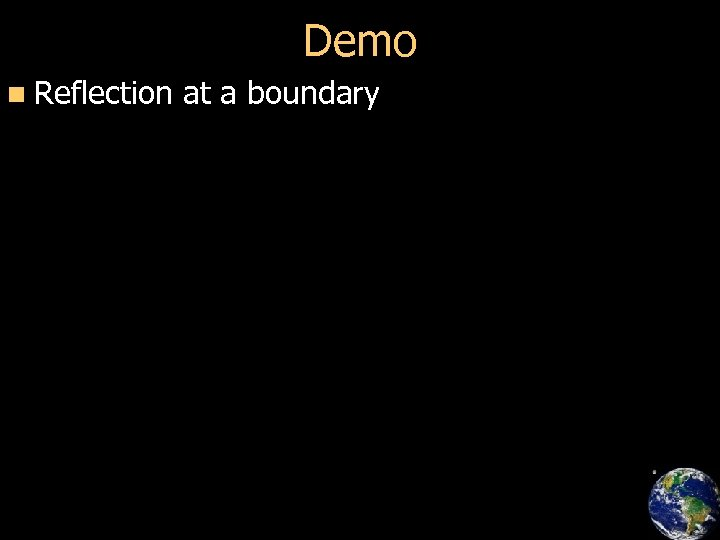Demo n Reflection at a boundary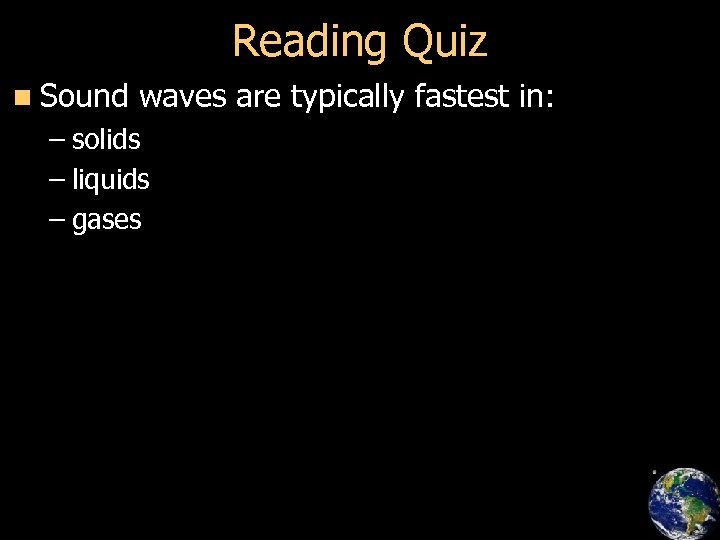Reading Quiz n Sound waves are typically fastest in: – solids – liquids – gases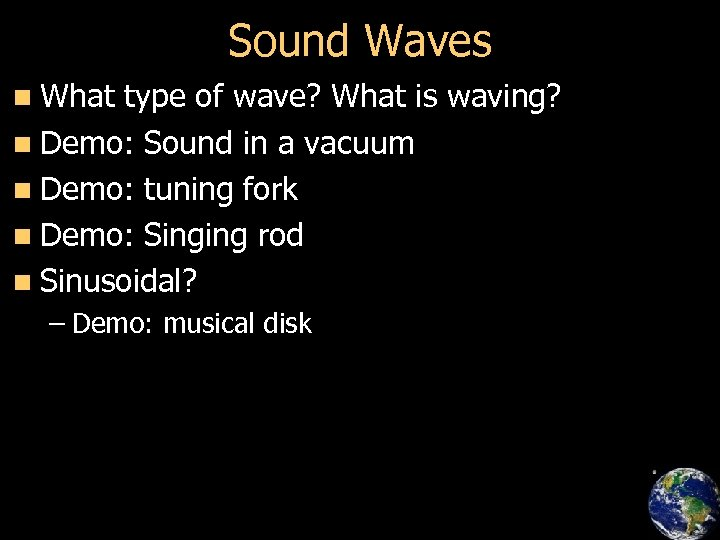Sound Waves n What type of wave? What is waving? n Demo: Sound in a vacuum n Demo: tuning fork n Demo: Singing rod n Sinusoidal? – Demo: musical disk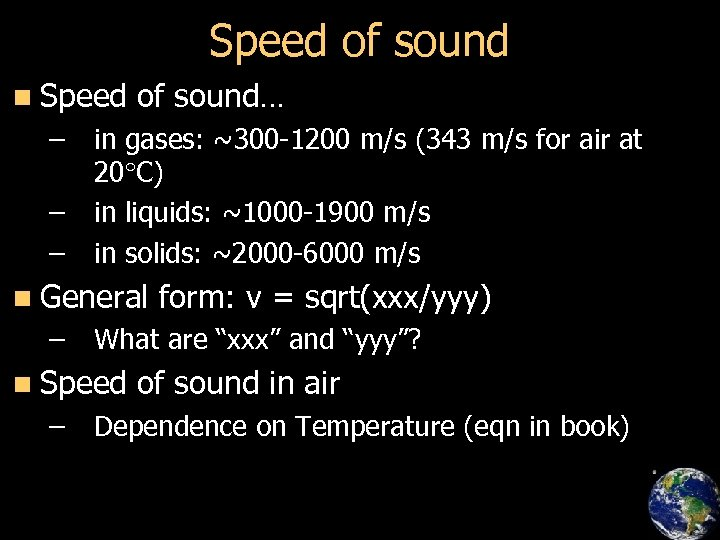Speed of sound n Speed – – – of sound… in gases: ~300 -1200 m/s (343 m/s for air at 20 C) in liquids: ~1000 -1900 m/s in solids: ~2000 -6000 m/s n General – What are “xxx” and “yyy”? n Speed – form: v = sqrt(xxx/yyy) of sound in air Dependence on Temperature (eqn in book)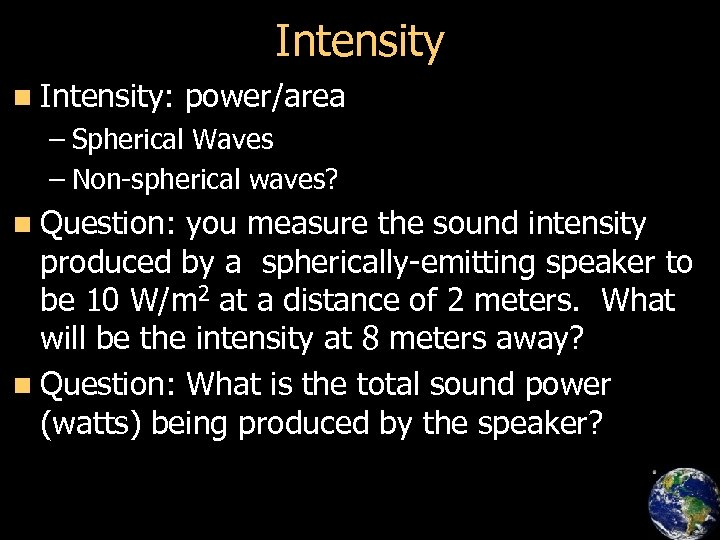Intensity n Intensity: power/area – Spherical Waves – Non-spherical waves? n Question: you measure the sound intensity produced by a spherically-emitting speaker to be 10 W/m 2 at a distance of 2 meters. What will be the intensity at 8 meters away? n Question: What is the total sound power (watts) being produced by the speaker?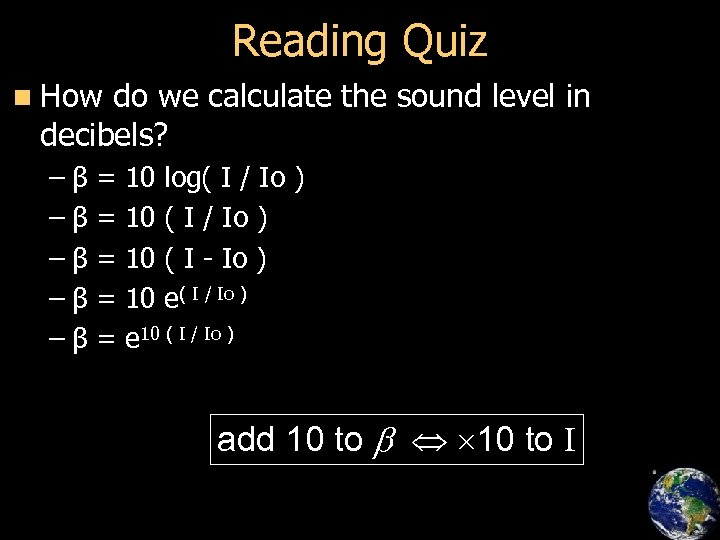Reading Quiz n How do we calculate the sound level in decibels? –β –β –β = = = 10 log( I / Io ) 10 ( I - Io ) 10 e( I / Io ) e 10 ( I / Io ) add 10 to b 10 to I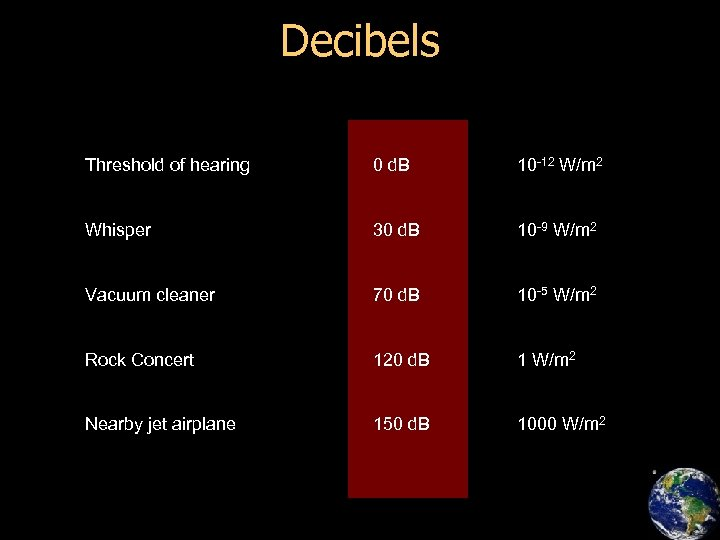Decibels Threshold of hearing 0 d. B 10 -12 W/m 2 Whisper 30 d. B 10 -9 W/m 2 Vacuum cleaner 70 d. B 10 -5 W/m 2 Rock Concert 120 d. B 1 W/m 2 Nearby jet airplane 150 d. B 1000 W/m 2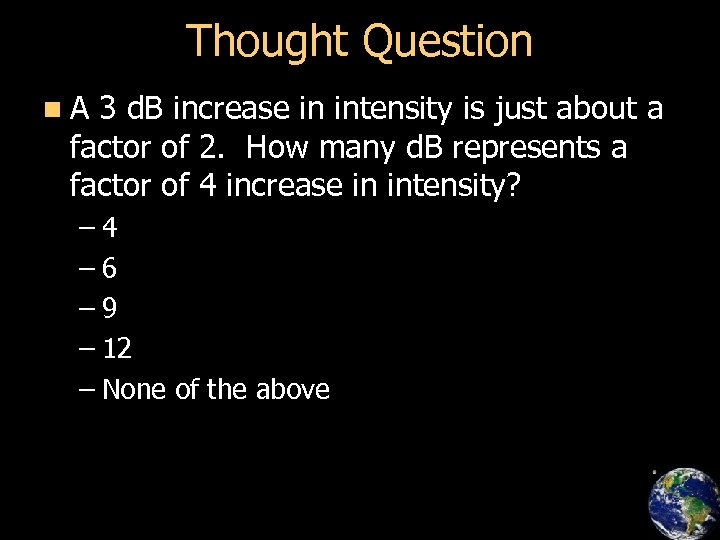Thought Question n. A 3 d. B increase in intensity is just about a factor of 2. How many d. B represents a factor of 4 increase in intensity? – 4 – 6 – 9 – 12 – None of the above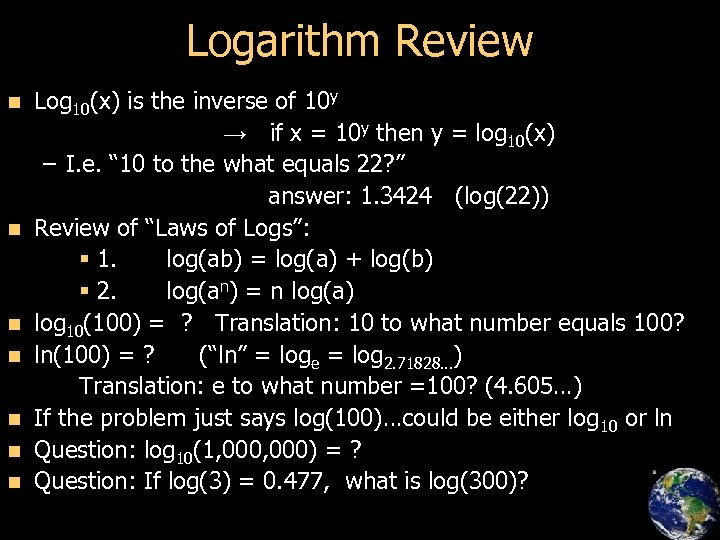Logarithm Review n n n n Log 10(x) is the inverse of 10 y → if x = 10 y then y = log 10(x) – I. e. “ 10 to the what equals 22? ” answer: 1. 3424 (log(22)) Review of “Laws of Logs”: § 1. log(ab) = log(a) + log(b) § 2. log(an) = n log(a) log 10(100) = ? Translation: 10 to what number equals 100? ln(100) = ? (“ln” = loge = log 2. 71828…) Translation: e to what number =100? (4. 605…) If the problem just says log(100)…could be either log 10 or ln Question: log 10(1, 000) = ? Question: If log(3) = 0. 477, what is log(300)?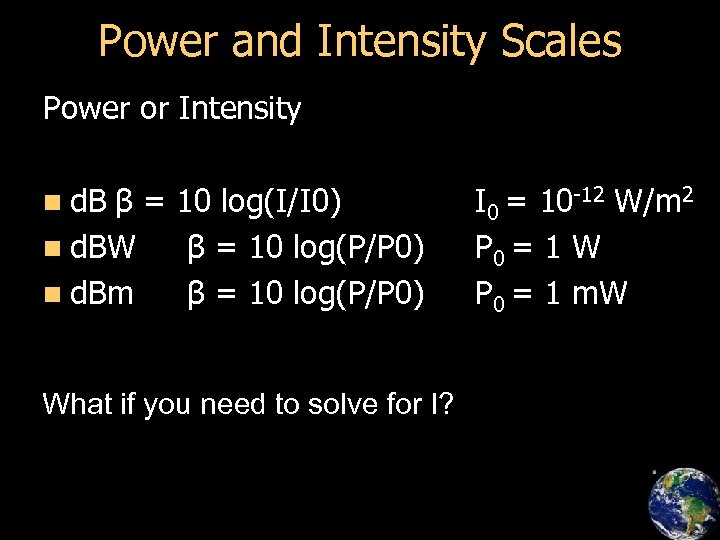Power and Intensity Scales Power or Intensity n d. B β = 10 log(I/I 0) n d. BW β = 10 log(P/P 0) n d. Bm β = 10 log(P/P 0) What if you need to solve for I? I 0 = 10 -12 W/m 2 P 0 = 1 W P 0 = 1 m. W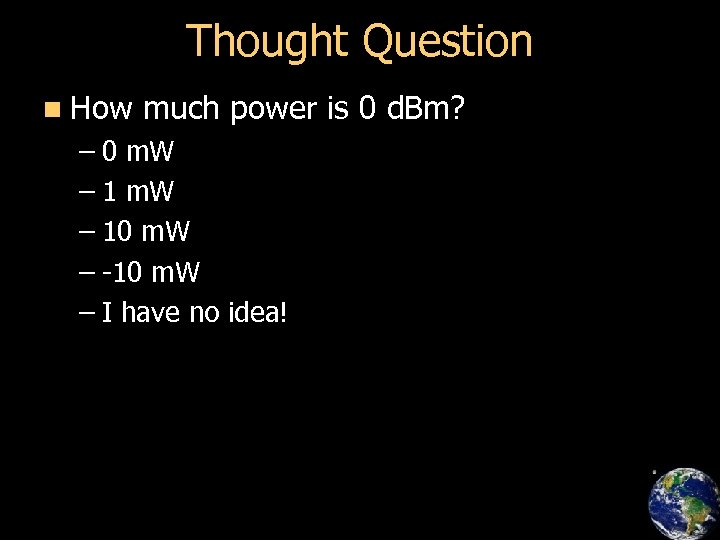Thought Question n How much power is 0 d. Bm? – 0 m. W – 10 m. W – -10 m. W – I have no idea!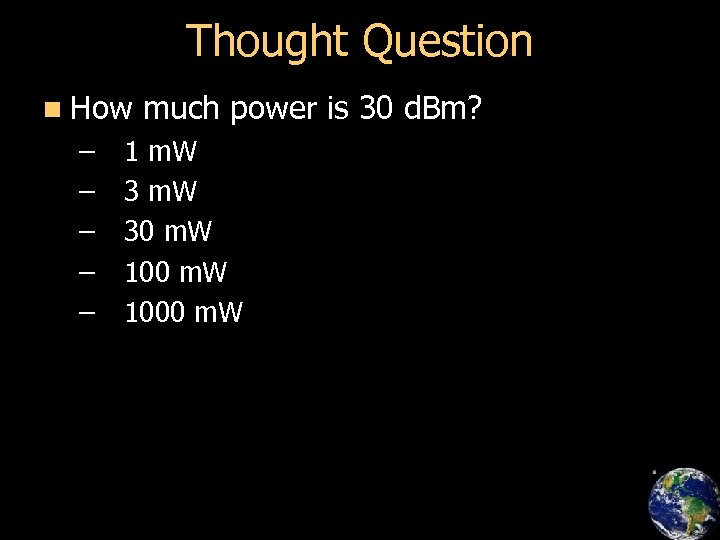Thought Question n How – – – much power is 30 d. Bm? 1 m. W 30 m. W 1000 m. W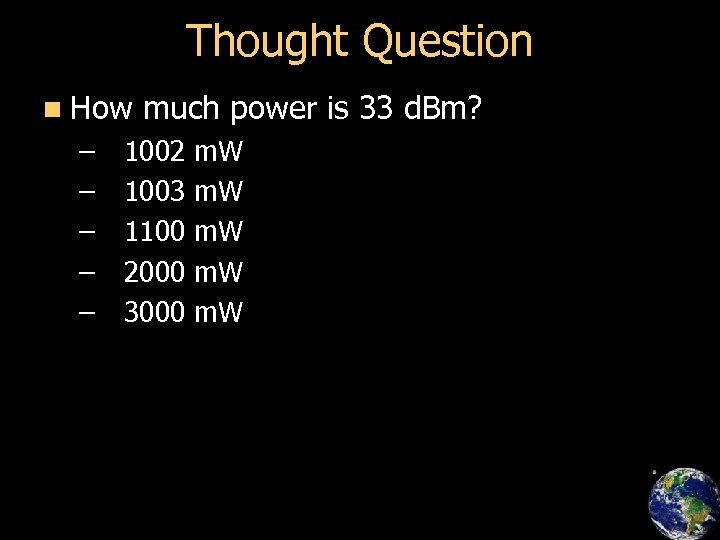Thought Question n How – – – much power is 33 d. Bm? 1002 1003 1100 2000 3000 m. W m. W